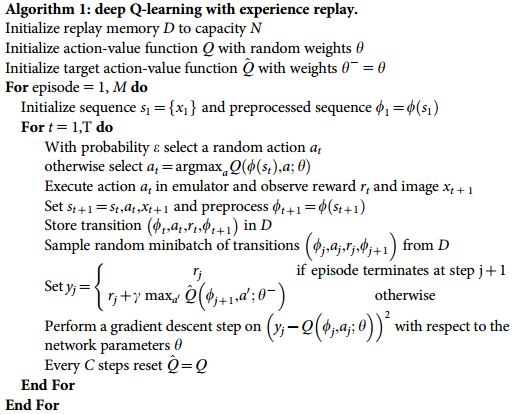# DQN 算法更新 (Tensorflow)

## 要点 ¶

Deep Q Network 的简称叫 DQN, 是将 Q learning 的优势 和 Neural networks 结合了. 如果我们使用 tabular Q learning, 对于每一个 state, action 我们都需要存放在一张 q_table 的表中. 如果像显示生活中, 情况可就比那个迷宫的状况复杂多了, 我们有千千万万个 state, 如果将这千万个 state 的值都放在表中, 受限于我们计算机硬件, 这样从表中获取数据, 更新数据是没有效率的. 这就是 DQN 产生的原因了. 我们可以使用神经网络来 估算 这个 state 的值, 这样就不需要一张表了.

## 算法 ¶• 记忆库 (用于重复学习)
• 神经网络计算 Q 值
• 暂时冻结 q_target 参数 (切断相关性)

## 算法的代码形式 ¶

from maze_env import Maze
from RL_brain import DeepQNetwork


def run_maze():
step = 0    # 用来控制什么时候学习
for episode in range(300):
# 初始化环境
observation = env.reset()

while True:
# 刷新环境
env.render()

# DQN 根据观测值选择行为
action = RL.choose_action(observation)

# 环境根据行为给出下一个 state, reward, 是否终止
observation_, reward, done = env.step(action)

# DQN 存储记忆
RL.store_transition(observation, action, reward, observation_)

# 控制学习起始时间和频率 (先累积一些记忆再开始学习)
if (step > 200) and (step % 5 == 0):
RL.learn()

# 将下一个 state_ 变为 下次循环的 state
observation = observation_

# 如果终止, 就跳出循环
if done:
break
step += 1   # 总步数

# end of game
print('game over')
env.destroy()

if __name__ == "__main__":
env = Maze()
RL = DeepQNetwork(env.n_actions, env.n_features,
learning_rate=0.01,
reward_decay=0.9,
e_greedy=0.9,
replace_target_iter=200,  # 每 200 步替换一次 target_net 的参数
memory_size=2000, # 记忆上限
# output_graph=True   # 是否输出 tensorboard 文件
)
env.after(100, run_maze)
env.mainloop()
RL.plot_cost()  # 观看神经网络的误差曲线